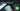# Hackerrank | Solution of Birthday Chocolate in Golang

October 4th, 2018
|

In this one we will solve question birthday chocolate from hackerrank in golang. Sweet one, let's dive right into it.

# Problem Statement

The question can be found at this link. The problem statement states that we are given a chocolate bar and two numbers. We need to find all those continuous length of chocolate bars where length of bar is equal to one number and sum of weights of bar is equal to other.

# Challenges

• Choose our preferred language as golang on hackerrank. The moment we do that, we get some 50-60 lines of code which are very unfamiliar to someone who is new to language.
• Cracking the logic required to solve the question.
• A chocolate bar nearby to celebrate once we solve the question

# Solution

This is the template you get on hackerrank for this problem statement.

``````    package main

import (
"bufio"
"fmt"
"io"
"os"
"strconv"
"strings"
)

// Complete the birthday function below.
func birthday(s []int32, d int32, m int32) int32 {

}

func main() {

stdout, err := os.Create(os.Getenv("OUTPUT_PATH"))
checkError(err)

defer stdout.Close()

writer := bufio.NewWriterSize(stdout, 16 * 1024 * 1024)

checkError(err)
n := int32(nTemp)

var s []int32

for i := 0; i < int(n); i++ {
sItemTemp, err := strconv.ParseInt(sTemp[i], 10, 64)
checkError(err)
sItem := int32(sItemTemp)
s = append(s, sItem)
}

dTemp, err := strconv.ParseInt(dm, 10, 64)
checkError(err)
d := int32(dTemp)

mTemp, err := strconv.ParseInt(dm, 10, 64)
checkError(err)
m := int32(mTemp)

result := birthday(s, d, m)

fmt.Fprintf(writer, "%d\n", result)

writer.Flush()
}

if err == io.EOF {
return ""
}

return strings.TrimRight(string(str), "\r\n")
}

func checkError(err error) {
if err != nil {
panic(err)
}
}``````
• First go ahead and delete everything in the pre-existing template.
• Let's write down our main wrapper function.
``````package main
import "fmt"

func main(){

}``````

Let's think about the variables we would need. We need the length of the bar `n`, the day `d` of birthday, the month of birthday `m` and the count of bar segments which satisfy the condition `c`. Now what about the array, do we need to take the whole array as input in an array or can we just keep taking the input in some temporary variable do some operation and be done with it ? In this case we need to loop over the bar segment after we have taken the input, so we need the array itself. Let's go ahead, declare the variables, array, and take the inputs as required.

``````	...
var n,d,m,c int
fmt.Scan(&n)

a:= make([]int,n)
for i:=0;i<n;i++{
fmt.Scan(&a[i])
}
fmt.Scan(&d,&m)``````

Here we have declared the variables, our array, and taken the inputs in the desired format. Now Let's discuss the logic. We need to start looking from the first block to last block. We also need an inner loop. We pick one block and start looping from there, adding the length of the bar as well as it's weight. The moment either the length or weight increases the month or day we break out of inner loop. The moment the length and weight equals month and day we found a match, we increase the counter and break out of loop. At the last we print the counter. If this sounds clear let's implement it.

``````    for i:=0;i<n;i++{
length,sum := 0,0
for j:=i;j<n;j++{
length++
sum += a[j]
if length == m && sum == d {
c++
break;
}
if sum > d || length > m{
break
}
}
// break brings here
}
fmt.Println(c)``````

So here we have an outer loop which will pick up the starting point of our bar. At the start we initialize the length and sum to `0`. Then we start looping from the `ith` position to end, till we find a match or condition fails. On each iteration, we increase the length of bar that we traversed and add the weight to sum. Then we apply our logic which we previously discussed. At the end we just print the counter. So now your complete code should look something like.

``````package main

import "fmt"

func main(){
var n,d,m,c int
fmt.Scan(&n)

a:= make([]int,n)
for i:=0;i<n;i++{
fmt.Scan(&a[i])
}
fmt.Scan(&d,&m)

for i:=0;i<n;i++{
length,sum := 0,0
for j:=i;j<n;j++{
length++
sum += a[j]
if length == m && sum == d {
c++
break;
}
if sum > d || length > m{
break
}
}
// break brings here
}
fmt.Println(c)
}``````

Now you can enjoy the chocolate bar I asked you keep nearby at the start of the article. This is it for this one, complete source code for this post can be found on my Github Repo. Will see you in the next one.